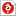검색어 입력폼

computer organization and architecture designing for performance 연습문제 13-15

저작시기 2017.12 |등록일 2019.03.31한컴오피스 (hwp) | 2페이지 | 가격 1,000원

소개글

computer organization and architecture designing for performance 연습문제 13-15

목차

1. Instruction size: 3 bytes, byte addressable, Integer: 8bit twos complement representation.

2. How many times does the processor need to refer to memory when it fetches and executes an instruction that requires computation of a single operand, if the addressing mode used is 1) indirect, 2) PC-relative.

3. A pipelined processor has a clock rate of 1GHz and executes a program with N instructions. The pipeline has five stages and instructions are issued at a rate of one per clock.

4. Explain the major characteristics common to all of RISC architectures.

5. Explain how CISC and RISC can reduce the program execution time(T) by managing which of Ic, CPI and τ

본문내용

1. Instruction size: 3 bytes, byte addressable, Integer: 8bit twos complement representation
R1 = 70, R2= 80, M = -16, M = -17, M = -18
300: MOV R2, #18 ; R2 <= 18
303: ADD R2, 1@R1 ; R2 <= R2 + M[R1+1]
306: BRAC \$-9 ; branch if carry (branch to –9@PC)

1) After executing MOV and ADD instructions, what value is stored in register R2?
Show the process of adding two binary numbers.

R2 = 18 – 17 = 0001 0010 + 1110 1111 = 0000 0001 = 1

2) Show the values of flags (C, AC, Z, S, OV) after ADD instruction.

C : 1
Z : 0
S : 0
OV : 0

없음